# ELG5103 Optical Communications Systems OZ Assignment Help## Question 1

1.Derive a formula that describes the responsivity as a function of vacuum wavelength of an ideal quantum detector.

The responsivity of a detector can be given by: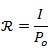where I is the photocurrent and Po is the incident optical power.

Further, we can define the quantum efficiency as: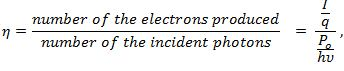Thus, this gives us the expression: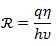And since,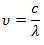So,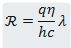(Senior, J, M, 2009)

2.Briefly, describe the processes in a practical pin-photodiode that cause the quantum efficiency to be less than unity and how considerations of maximizing the quantum efficiency and speed of response influences its structure.

The quantum efficiency can be less than unity in a p-i-n photodiode because the detector of the photodiode may be unable to receive all the incident photons and thus subsequently it would not be able to convert them all into the electron-hole pairs. To maximize the quantum efficiency and the seed of the response, the detector’s thickness should be kept broad so as to collect the incident photons properly. It should be kept greater than 0.5 cm for doped Ge and greater than 0.1 for doped Si. The speed of response also affects the structure of the photodiode as the junction capacitance gets reduced when the depletion layer is widened.

3.Calculate the ideal responsivity of a InGaAs (energy gap Eg = 0.75eV) photodiode when operating with incident light of vacuum wavelength:

a.λ=1550nm;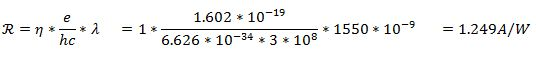b.λ=1700nm.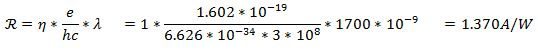### Question 2

1.List and briefly describe the main sources of noise encountered when measuring an optical signal. Give relevant formulae and suggest remedies where applicable.

The main sources of noise encountered when measuring an optical signal are:

1.Johnson Noise:

The noise voltage is given by,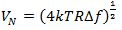2.Generation-Recombination Noise:

This arises due to a number of reasons which are,

a.Fluctuation in background radiation

The RMS fluctuation is given by: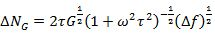b.Fluctuation in thermally generated electrons

The RMS fluctuation is given by: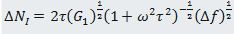c.Fluctuation in impact ionization rates

The RMS fluctuation is given by: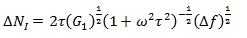The remedies for the noises generated can be the limiting of bandwidth by using a filter circuit, or by choosing an amp with lower voltage, or by reducing the RF by choosing a more sensitive diode.

2.A high-speed pin-photodiode a responsivity of R=1A/W and capacitance C=1pF operates in the photoconductive mode into a resistive load RL=50Ω . Calculate the bandwidth and sensitivity of the resulting photoreceiver circuit at a temperature T=300K.

Bandwidth,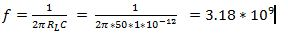Sensitivity=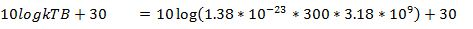=-108.80+30   =-78.80dBm

(Layne D., 2017)

3.The same photodiode is connected to a transimpedance amplifier with an open-loop gain of A=99  and feedback resistor RF=1kΩ . Find the new value of sensitivity assuming a noise-free wideband amplifier.

Bandwidth =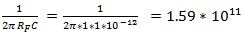Sensitivity=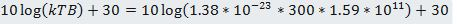=-91.82+30   =-61.82dBm.

4.The feedback resistor is found to have a shunt capacitance of 200fF. How does this affect your results?

The bandwidth will change to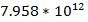and the sensitivity will change to -44.82dBm .

5.Describe the options available and their practicality to improve sensitivity towards the quantum limit.

The options that can be used to improve the sensitivity are by using the active techniques instead of the resistive loading. It involves either feeding the photocurrent into an active circuit or putting the current gain into the detector. Further, it may also be improved by using either a coherent detection or by using an optical amplifier which would make the optical signal stronger before it is detected.

The active techniques are feasible but making the signal stronger may employ the devices posing a problem of spatial arrangements or costs of operating them.

### Question 3

1.Provide a physical justification of a simple equivalent circuit of an ideal pin photodiode specifying the I-V characteristics of its principal elements.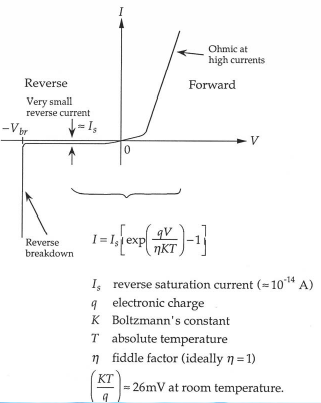The principal elements of a p-i-n photodiode are the p-type layer, intrinsic layer and the n-type layer. The intrinsic layer provides a great reverse-breakdown voltage and a low capacitance level. At reverse bias, the layer becomes fully depleted at low levels. At forward bias, it shows ohmic behavior at high levels.

2.Use your equivalent circuit to derive an equation for the voltage V delivered by a photodiode operating in the photovoltaic mode to a load drawing a current I at an incident optical power Po. Use your result to find expressions for short-circuit current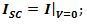the open circuit voltage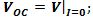the product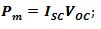the electrical power delivered to the load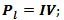and the power ratio: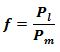Using the equivalent circuit, the equations for the above may be represented as:

The short circuit current is the one obtained when the potential difference across the nodes through which the current is calculated is zero.

Short-circuit current: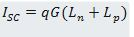The open circuit voltage is the one obtained when the current flowing between the two nodes becomes zero, or it may said that the resistance between the two has become infinite.

Open circuit voltage: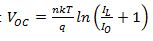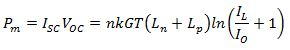Power ratio: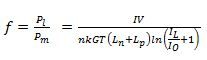3.This ratio depends upon the incident optical power Po through the photo-generated current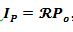, where R is the responsivity (found in Question 1) and on the current drawn by the load I. These currents are conveniently normalized by the dark reverse saturation current IS of the ideal photodiode: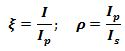The parameter ρis very large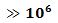for practical values of photocurrents and reverse saturation currents.

Use Matlab to plot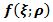versus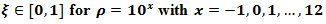to illustrate that: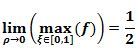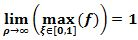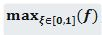is a monotone increasing function of ρThe graph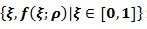for large ρapproaches a straight-line of unit slope.

In the photovoltaics community,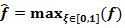is known as ‘fill factor’.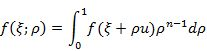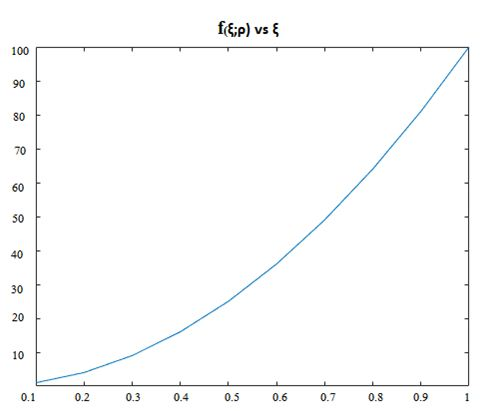The graph plotted between the two entities shows a constant increase in the value between the two entities. As the value of ρincreases towards large values, the graph takes up a constant increasing shape of slope being unity.

4.Piece your results together to give an expression for a critical optical power Po for which the electrical power Pl delivered to the load equals the optical power Po incident on the photodiode. It follows that the model predicts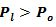for optical powers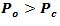in violation of the 1st law of thermodynamics.

Given an explanation of the reason for the failure of the model (Hint: Evaluate the potential energy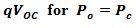making use of the expression for the ideal responsivity derived in Question 1 and compare with the energy gap of the semiconductor).

The first law of thermodynamics or the law of conservation of energy states that the total energy of a system remains constant in an isolated system and the energy may just transfer from on to another form. The model here displays the photons displaying energy such that the electrical power of the load is greater than the optical power. But, since the first law states that energy will be constant, the model therefore violates the first law.

5.In addition to the ‘heat’ input from a hot reservoir (the incident optical power) and the work out (electrical power delivered to the load), the 2nd law of thermodynamics requires a ‘heat’ output to a cool reservoir (the ambient). Describe the physical processes responsible for converting the excess energy of the photon into this heat output.

There is a heat addition from a hot reservoir in form of the incident optical power and then the work is done in the form of the power delivered to the load. This alone doesn’t satisfy the second law of thermodynamics and therefore requires a heat output to a cool reservoir which can be seen as the heat emitted by the semiconductor the surrounding.

### Question 4

This question concerns a fiber optic link operating at a bit rate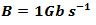using a standard fiber with a dispersion of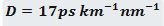and an attenuation α=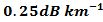at a vacuum wavelength λ=1.55μm , and a zero dispersion fiber (D=0) with an attenuation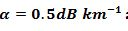at a vacuum wavelength λ=1.3μm.

1.Briefly describe the main sources of attenuation and dispersion in an optical fiber data link and how the limitations these place on performance may be alleviated.

Attenuation is the process of optical power loss in the light intensity when it passes through an optical fiber cable. The main sources for attenuation are absorption (by conversion of optical power into another form such as heat), scattering (i.e., due to density variation in the fiber) and bending losses (due to bending of the fiber). The dispersion in optical fiber occurs due to the spreading of the light beams when they travel through the fiber. An index profile of nearly parabolic shape may be incorporated to alleviate this.

2.You have a transmitter that operates at a vacuum wavelength λ=1.55μm , has a spectral width ?λ=1nm , and an output power of 10 mW. The receiver requires –20 dBm of input power to achieve the desired bit error rate. What is the length of the longest link you can build?

Length of the link,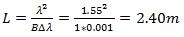3.You have another transmitter that operates at a vacuum wavelength of λ=1.3μm , has a spectral width ?λ=2nm  and an output power of 1 mW. Assume the same receiver as before. What is the length of the longest link you can build?

Length of the link,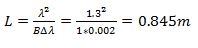### Question 5

Consider a standard double heterojunction laser diode. Well below threshold this device operates as a light-emitting diode, whereas well above threshold it behaves as a laser. Why is the overall power conversion efficiency (emitted light power leaving the device divided by electrical power) typically much better for laser operation than for LED-operation?

The power conversion efficiency is much better for the laser operation than for the LED-operation because in the light emitting diodes, there is little or no polarization as well as a high NA. There is a poor coupling into the fiber due to which the light intensity being transmitted gets distorted. While a LASER on the other hand is more polarized and thus, it displays better power conversion efficiency.

### References

1. Layne, Denis. "Receiver Sensitivity And Equivalent Noise Bandwidth". Highfrequencyelectronics.com. N.p., 2017. Web. 30 Mar. 2017.

2. Senior, John M. Optical Fiber Communications Principles And Practice. 3rd ed. Harlow: Pearson Education Limited, 2017. Print.